Courses

# Test: Methods of Indeterminate Analysis -2

## 10 Questions MCQ Test Topicwise Question Bank for Civil Engineering | Test: Methods of Indeterminate Analysis -2

Description
This mock test of Test: Methods of Indeterminate Analysis -2 for Civil Engineering (CE) helps you for every Civil Engineering (CE) entrance exam. This contains 10 Multiple Choice Questions for Civil Engineering (CE) Test: Methods of Indeterminate Analysis -2 (mcq) to study with solutions a complete question bank. The solved questions answers in this Test: Methods of Indeterminate Analysis -2 quiz give you a good mix of easy questions and tough questions. Civil Engineering (CE) students definitely take this Test: Methods of Indeterminate Analysis -2 exercise for a better result in the exam. You can find other Test: Methods of Indeterminate Analysis -2 extra questions, long questions & short questions for Civil Engineering (CE) on EduRev as well by searching above.
QUESTION: 1

### A horizontal fixed beam AB of span 6 m has uniform flexural rigidity of 4200 kN m2. During loading, the support B sinks downwards by 25 mm. The moment induced at the end A is

Solution: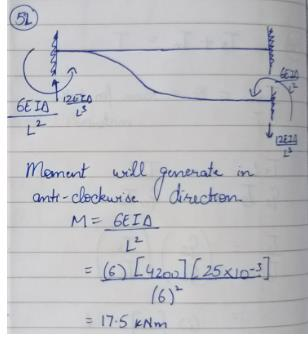QUESTION: 2

### A uniform simply supported beam is subjected to a clockwise moment M at the left end. What is the moment required at the right end so that rotation of the right end is zero?

Solution: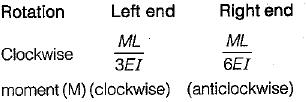at left end
To keep rotation at right and zero a moment should be applied in anticlockwise direction. Let the moment in M'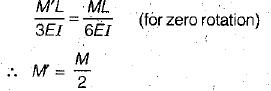QUESTION: 3

### The possible direction of sway of the rigid frame shown in figure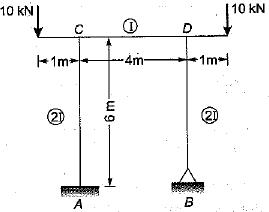Solution:
QUESTION: 4

Four identical beams AE, BE, CE and DEhave been rigidly jointed at E The point O slip and rotates along with member firmly fixed at E.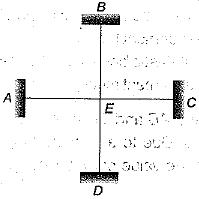Which one among the following is correct?

Solution:

Since the member are firmly fixed at E. It is a rigid joint at which all the members will have same rotation. So from slope deflection equations all the members will have moment.

QUESTION: 5

Match List-I (Method) with List-lI (Factor) and select the correct answer using the code given below the lists:
List-I
A. Moment distribution method
B. Slope deflection method
C. Kani’s method
D. Force method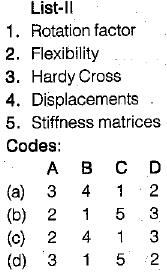Solution:

A -3 , B -4 , C - 1 , D - 2

QUESTION: 6

Final moment values got through the analysis of a portal frame have been shown in the figure. What is the value of X?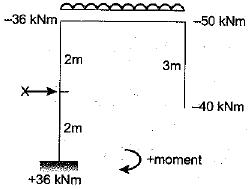Solution: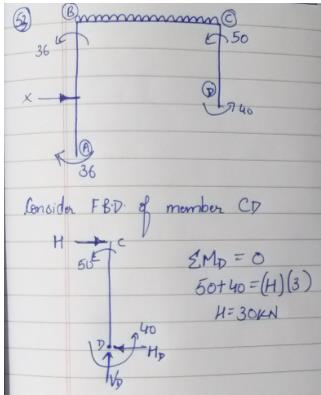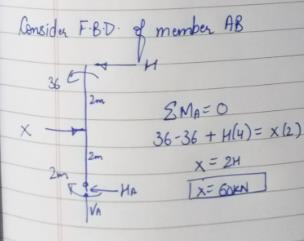QUESTION: 7

The figure given above shows a rigid frame. If D . is lateral translation of the joints, slope deflection equation for the member BA be written as: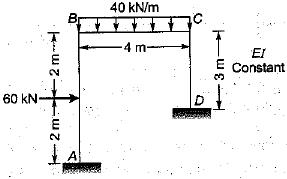Solution:

Taking;
- anticlockwise end moment positive
- anticlockwise rotation at member end positive
- anticlockwise chord rotation positive
FEMba = 30 kN-m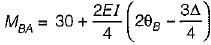QUESTION: 8

What are the distribution factors at joint 6 for the members BA and BC respectively, in the figure?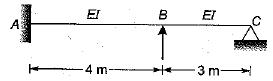Solution:

Distribution Factors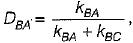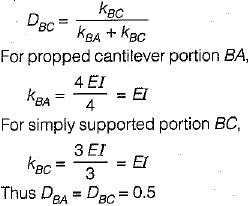QUESTION: 9

Which one of the following statements is correct? The principle of superposition is applicable to

Solution:

The principle of superposition states that the displacements resulting from each of a number of forces may be added to obtain the displacements resulting from the sum of forces. This method depends upon the linearity of the governing relations between the load and deflection, The linearity depends upon two factors.
(i) the linearity between bending moment and curvature which depends upon the linear elastic materials  In non-linear material superposition of curvatures is not possible.
(ii) the linearity between curvatures and deflection depends upon the assumption that the deflections are so small that the approximate curvature can be used in place of true curvature.

QUESTION: 10

Members AB and BC in the figure shown are identical. Due to a moment 2M applied at B, what is the value of axial force in the member AB?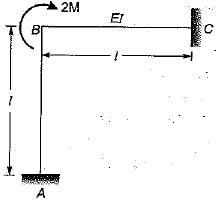Solution:

The distribution factors for member AB and SC at S will be 0.5 for both.
Thus member end moment at BC is M.
From carry over factor of 1/2, member end moment at C = M/2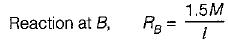This reaction is away from B so axial force in member AB is tensile. This can be found from free body diagram of AB. Similarly axial force in member BC will also be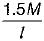but compressive.